# Chapter 4 Symbolizing features

## 4.1 Color

Each color is a combination of three perceptual dimensions: hue, lightness and saturation.

### 4.1.1 Hue

Hue is the perceptual dimension associated with color names. Typically, we use different hues to represent different categories of data.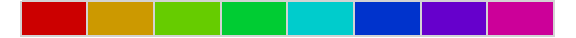Figure 4.1: An example of eight different hues. Hues are associated with color names such as green, red or blue.

Note that magentas and purples are not part of the natural visible light spectrum; instead they are a mix of reds and blues (or violets) from the spectrum’s tail ends.

### 4.1.2 Lightness

Lightness (sometimes referred to as value) describes how much light reflects (or is emitted) off of a surface. Lightness is an important dimension for representing ordinal/interval/ratio data.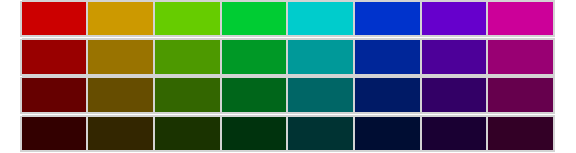Figure 4.2: Eight different hues (across columns) with decreasing lightness values (across rows).

### 4.1.3 Saturation

Saturation (sometimes referred to as chroma) is a measure of a color’s vividness. You can use saturated colors to help distinguish map symbols. But be careful when manipulating saturation, its property should be modified sparingly in most maps.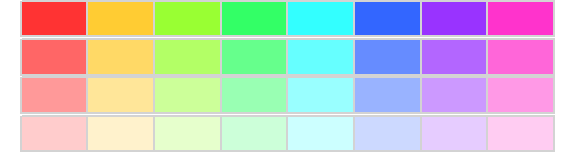Figure 4.3: Eight different hues (across columns) with decreasing saturation values (across rows).

## 4.2 Color Space

The three perceptual dimensions of color can be used to construct a 3D color space. This 3D space need not be a cube (as one would expect given that we are combining three dimensions) but a cone where lightness, saturation and hue are the cone’s height, radius and circumference respectively.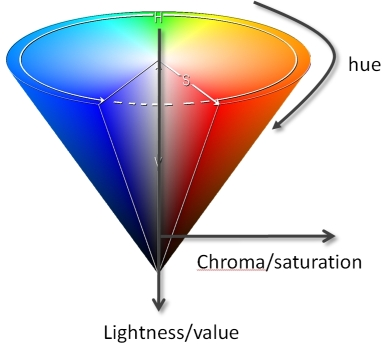Figure 4.4: This is how the software defines the color space. But does this match our perception of color space?

The cone shape reflects the fact that as one decreases saturation, the distinction between different hues disappears leading to a grayscale color (the central axis of the cone). So if one sets the saturation value of a color to 0, the hue ends up being some shade of grey.

The color space implemented in most software is symmetrical about the value/lightness axis. However, this is not how we “perceive” color space: our perceptual view of the color space is not perfectly symmetrical.

Let’s examine a slice of the symmetrical color space along the blue/yellow hue axis at a lightness value of about 90%.Figure 4.5: A cross section of the color space with constant hues and lightness values and decreasing saturation values where the two hues merge.

Now, how many distinct yellows can you make out? How many distinct blues can you make out? Do the numbers match? Unless you have incredible color perception, you will probably observe that the number of distinct colors do not match when in fact they do! There are exactly 30 distinct blues and 30 distinct yellows. Let’s add a border to each color to convince ourselves that the software did indeed generate the same number of distinct colors.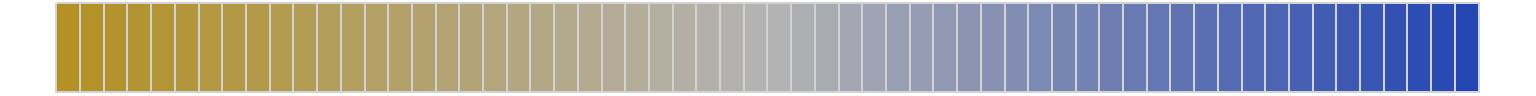Figure 4.6: A cross section of the color space with each color distinctly outlined.

It should be clear by now that a symmetrical color space does not reflect the way we “perceive” colors. There are more rigorously designed color spaces such as CIELAB and Munsell that depict the color space as a non-symmetrical object as perceived by humans. For example, in a Munsell color space, a vertical slice of the cone along the blue/yellow axis looks like this.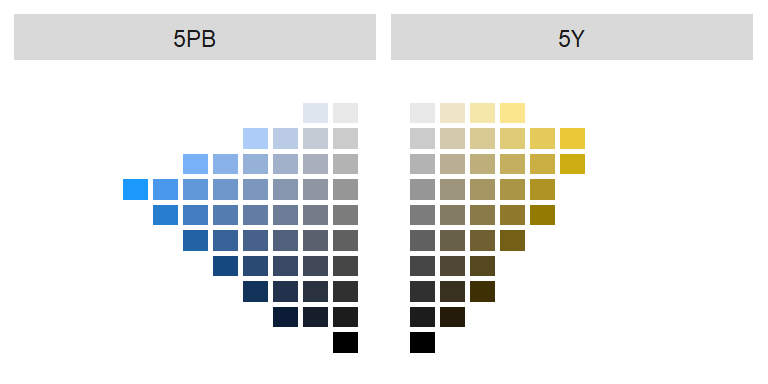Figure 4.7: A slice of the Munsell color space.

Note that based on the Munsell color space, we can make out fewer yellows than blues across all lightness values. In fact, for these two hues, we can make out only 29 different shades of yellow (we do not include the gray levels where saturation = 0) vs 36 shades of blue.

So how do we leverage our understanding of color spaces when choosing colors for our map features? The next section highlights three different color schemes: qualitative, sequential and divergent.

## 4.3 Classification

### 4.3.1 Qualitative color scheme

Qualitative schemes are used to symbolize data having no inherent order (i.e. categorical data). Different hues with equal lightness and saturation values are normally used to distinguish different categorical values.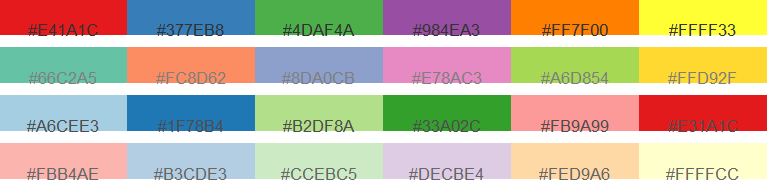Figure 4.8: Example of four different qualitative color schemes. Color hex numbers are superimposed on each palette.

Election results is an example of a dataset that can be displayed using a qualitative color scheme. But be careful in your choice of hues if a cultural bias exists (i.e. it may not make sense to assign “blue” to republican or “red”” to democratic regions).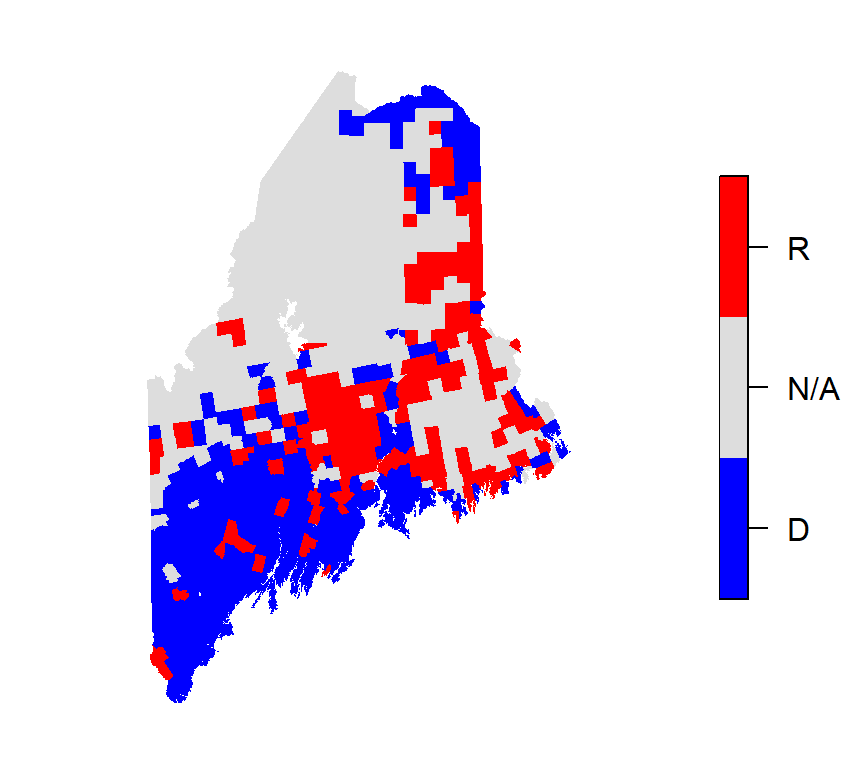Figure 4.9: Map of 2012 election results shown in a qualitative color scheme. Note the use of three hues (red, blue and gray) of equal lightness and saturation.

Most maps created in this course will be generated from polygon layers where continuous values will be assigned discrete color swatches. Such maps are referred to as choropleth maps. The choice of classification schemes for choropleth maps are shown next.

### 4.3.2 Sequential color scheme

Sequential color schemes are used to highlight ordered data such as income, temperature, elevation or infection rates. A well designed sequential color scheme ranges from a light color (representing low attribute values) to a dark color (representing high attribute values). Such color schemes are typically composed of a single hue, but may include two hues as shown in the last two color schemes of the following figure.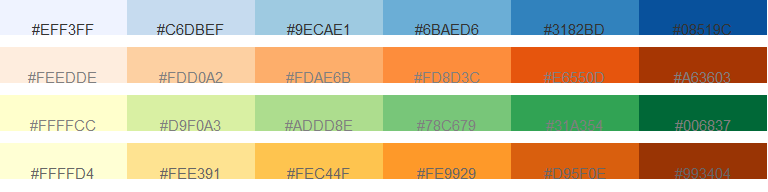Figure 4.10: Example of four different sequential color schemes. Color hex numbers are superimposed on each palette.

Distribution of income is a good example of a sequential map. Income values are interval/ratio data which have an implied order.Figure 4.11: Map of household income shown in a sequential color scheme. Note the use of a single hue (green) and 7 different lightness levels.

### 4.3.3 Divergent color scheme

Divergent color schemes apply to ordered data as well. However, there is an implied central value about which all values are compared. Typically, a divergent color scheme is composed of two hues–one for each side of the central value. Each hue’s lightness/saturation value is then adjusted symmetrically about the central value. Examples of such a color scheme follows: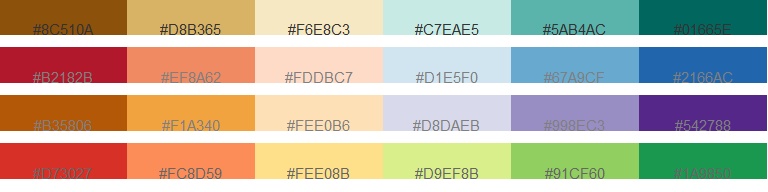Figure 4.12: Example of four different divergent color schemes. Color hex numbers are superimposed onto each palette.

Continuing with the last example, we now focus on the divergence of income values about the median value of \$36,641. We use a brown hue for income values below the median and a green/blue hue for values above the median.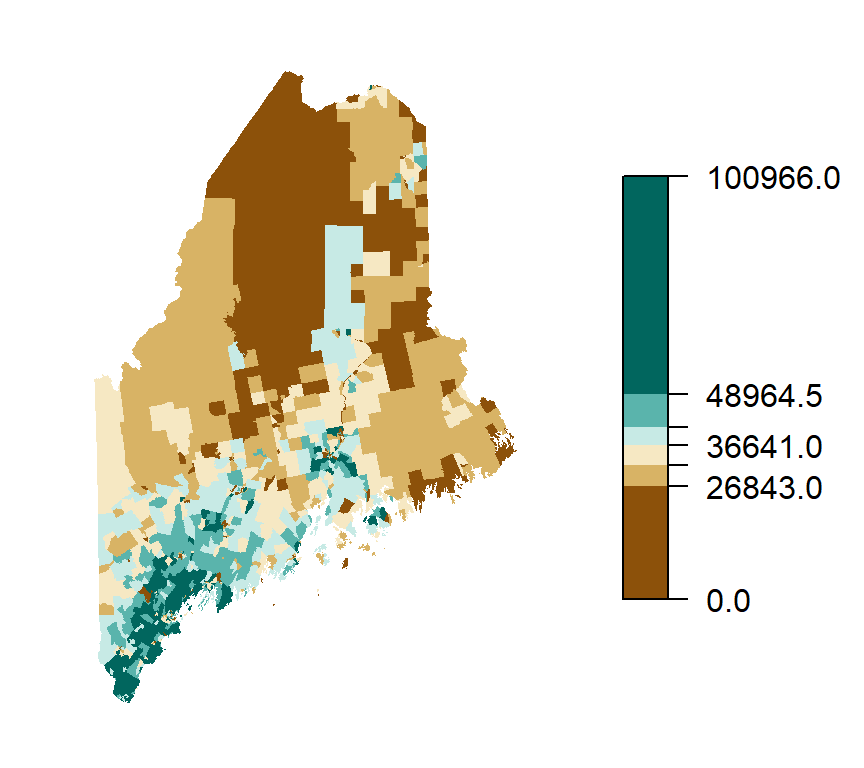Figure 4.13: This map of household income uses a divergent color scheme where two different hues (brown and blue-green) are used for two sets of values separated by the median income of 36,641 dollars. Each hue is then split into three separate colors using decreasing lightness values away from the median.

## 4.4 So how do I find a proper color scheme for my data?

Fortunately, there is a wonderful online resource that will guide you through the process of picking a proper set of color swatches given the nature of your data (i.e. sequential, diverging, and qualitative) and the number of intervals (aka classes). The website is http://colorbrewer2.org/ and was developed by Cynthia Brewer et. al at the Pennsylvania State University.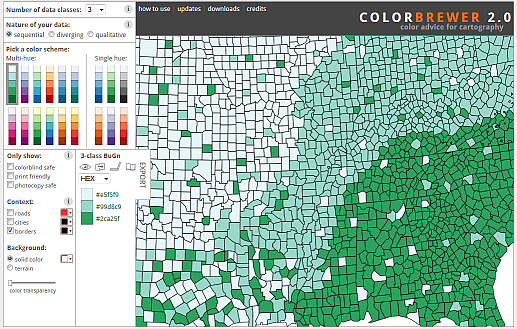You’ll note that the ColorBrewer website limits the total number of color swatches to 12 or less. There is a good reason for this in that our eyes can only associate so many different colors with value ranges/bins. Try matching 9 different shades of green in a map to the legend box swatches!

Additional features available on that website include choosing colorblind safe colors and color schemes that translate well into grayscale colors (useful if your work is to be published in journals that do not offer color prints).

## 4.5 Classification Intervals

You may have noticed the use of different classification breaks in the last two maps. For the sequential color scheme map, an equal interval classification scheme was used where the full range of values in the map are split equally into 7 intervals so that each color swatch covers an equal range of values. The divergent color scheme map adopts a quantile interval classification where each color swatch is represented an equal number of times across each polygon.

Using different classification intervals will result in different looking maps. In the following figure, three maps of household income (aggregated at the census tract level) are presented using different classification intervals: quantile, equal and Jenks. Note the different range of values covered by each color swatch.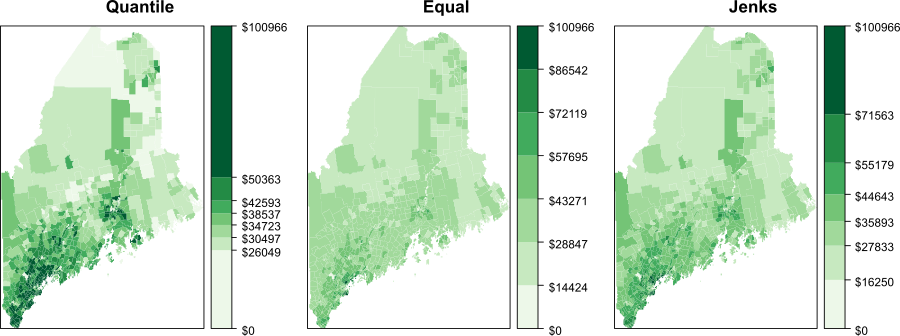Figure 2.3: Three different representations of the same spatial data using different classification intervals.

The quantile interval scheme ensures that each color swatch is represented an equal number of times. If we have 20 polygons and 5 classes, the interval breaks will be such that each color is assigned to 4 different polygons.

The equal interval scheme breaks up the range of values into equal interval widths. If the polygon values range from 10,000 to 25,000 and we have 5 classes, the intervals will be [10,000 ; 13,000], [13,000 ; 16,000], …, [22,000 ; 25,000].

The Jenks interval scheme (aka natural breaks) uses an algorithm that identifies clusters in the dataset. The number of clusters is defined by the desired number of intervals.

It may help to view the breaks when superimposed on top of a distribution of the attribute data. In the following graphics the three classification intervals are superimposed on a histogram of the per-household income data. The histogram shows the distribution of values as “bins” where each bin represents a range of income values. The y-axis shows the frequency (or number of occurrences) for values in each bin.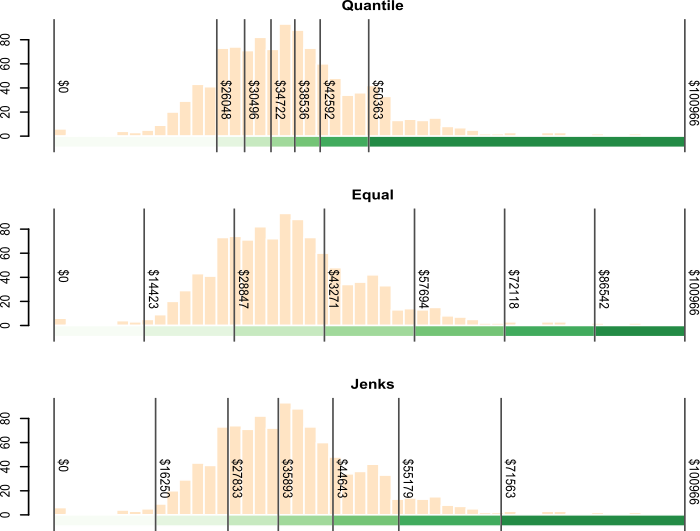Figure 4.14: Three different classification intervals used in the three maps. Note how each interval scheme encompasses different ranges of values (hence the reason all three maps look so different).

### 4.5.1 An Interactive Example

The following interactive frame demonstrates the different “looks” a map can take given different combinations of classification schemes and class numbers.